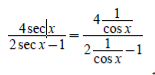• FAQ
• Contact/ Homework Answers / Mathematics / Prepare for Section 8.6 P1. Find the eccentricity of the graph. x2/25
Not my Question
Flag Content

# Question

Prepare for Section 8.6

P1. Find the eccentricity of the graph.

x2/25 + y2/16  =1

P2. Find the equation of the directrix.

y2 = 4x

P3. Solve for y.

y = 2(1+ yx)

P4. Find the smallest value of x excluded from the domain.

1+sin x = 0

P6. Write in terms of cos x.## Solution 5 (1 Ratings )

Solved
Mathematics 1 Month Ago 7 Views